# System Of Linear Equations JEE Notes | EduRev

## JEE : System Of Linear Equations JEE Notes | EduRev

The document System Of Linear Equations JEE Notes | EduRev is a part of the JEE Course Mathematics (Maths) Class 12.
All you need of JEE at this link: JEE

I. SYSTEM OF LINEAR EQUATIONS
System Of Linear Equation (In Two Variables) :
(i) Consistent Equations :       Definite & unique solution .       [ intersecting lines ]
(ii) Inconsistent Equation :      No solution .                            [ Parallel line ]
(iii) Dependent equation :        Infinite solutions .                    [ Identical lines ]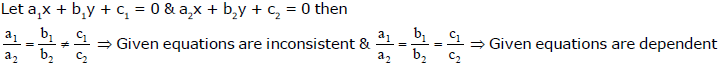Cramer's Rule :[ Simultaneous Equations Involving Three Unknowns ]
Let a1x + b1y + c1z = d1 ..... (I) ;

a2x + b2y + c2z = d2 ..... (II) ;

a3x + b3y + c3z = d3 ..... (III)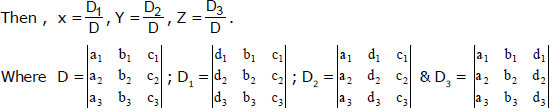Note :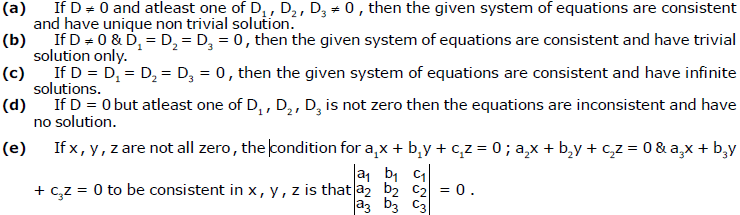Remember that if a given system of linear equations have Only Zero Solution for all its variables then
the given equations are said to have Trivial Solution.

Solving System of Linear Equations Using Matrices :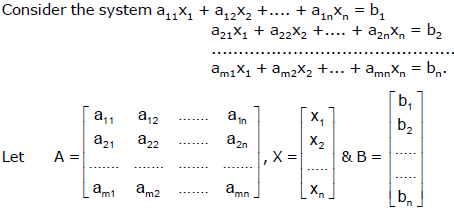Then the above system can be expressed in the matrix form as AX = B.
The system is said to be consistent if it has atleast one solution.

(i) System of Linear Equations And Matrix Inverse :
If the above system consist of n equations in n unknowns, then we have AX = B where A is a
square matrix. If A is non–singular, solution is given by X = A–1B.
If A is singular, (adj A) B = 0 and all the columns of A are not proportional, then the system has
infinitely many solutions.
If A is singular and (adj A) B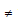0, then the system has no solution (we say it is inconsistent).

(ii) Homogeneous System and Matrix Inverse :
If the above system is homogeneous, n equations in n unknowns, then in the matrix form it is
AX = O. (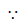in this case b1 = b2 =..... bn = 0), where A is a square matrix.
If A is non–singular, the system has only the trivial solution (zero solution) X = 0
If A is singular, then the system has infinitely many solutions (including the trivial solution) and hence it has non–trivial solutions.

(iii) Elementary Row Transformation of Matrix :
The following operations on a matrix are called as elementary row transformations.
(a) Interchanging two rows.
(b) Multiplications of all the elements of row by a nonzero scalar.
(c) Addition of constant multiple of a row to another row.

Note : Similar to above we have elementary column transformations also.

Remark : Two matrices A & B are said to be equivalent if one is obtained from other using elementary
transformations. We write A ~ B.

(iv) Echelon Form of A Matrix : A matrix is said to be in Echelon form if it satisfies the following
(a) The first non-zero element in each row is 1 & all the other elements in the corresponding
column (i.e. the column where 1 appears) are zeroes.
(b) The number of zeros before the first non zero element in any non zero row is less than
the number of such zeroes in succeeding non zero rows.

(v) System of Linear Equations : Let the system be AX = B where A is an m × n matrix, X is
the n–column vector & B is the m–column vector. Let [AB] denote the augmented matrix (i.e.
matrix obtained by accepting elements of B as n + 1th column & first n columns are that of A).

Ex.25 Solve the equations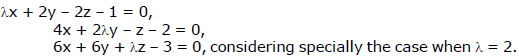Sol.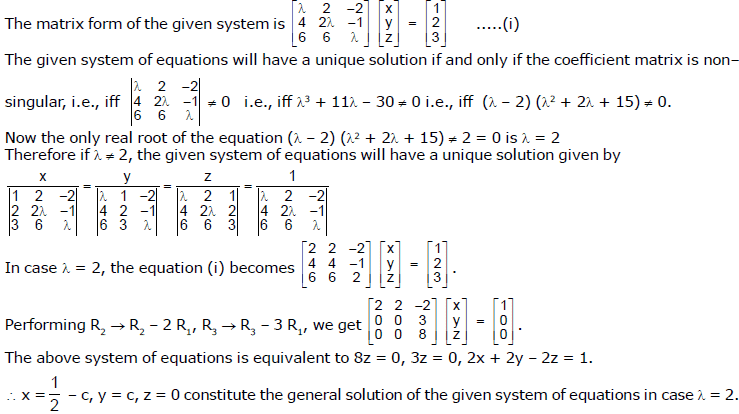Ex.26 Solve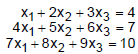Sol.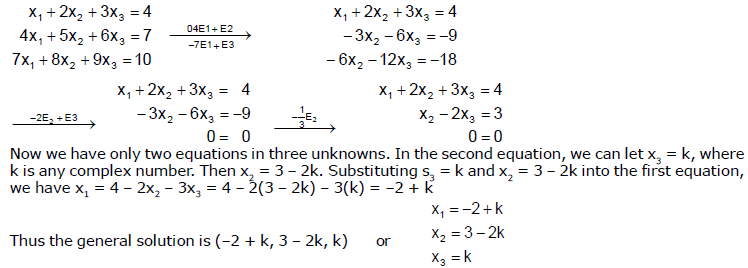And we see that the system has an infinite number of solutions. Specific solutions can be generated by
choosing specific values for k.

Ex.27 Number of triplets of a, b & c for which the system of equations ax - by = 2a - b and (c + 1)x + cy = 10 - a + 3 b has infinitely many solutions and x = 1, y = 3 is one of the solutions is

Sol.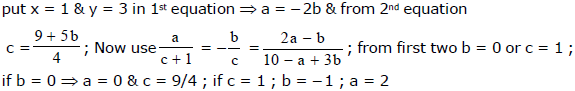Ex.28 Solve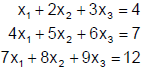Sol.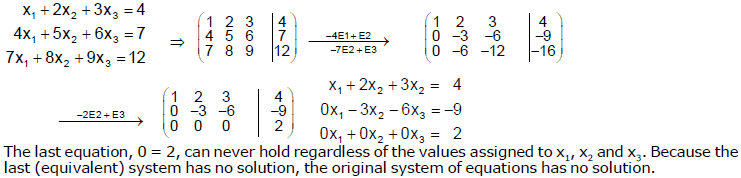Ex.29 Solve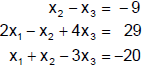by reducing the augmented matrix of the system to reduced row echelon form.

Sol.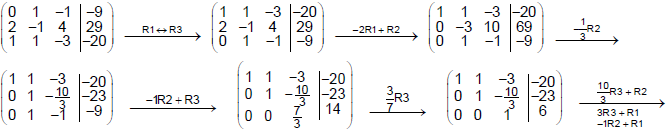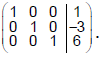It is easy to see that x1 = 1, x2 = –3, x3 = 6. The process of solving a system by reducin
the augmented matrix to reduced row echelon form is called Gauss–Jordan elimination.

Ex.30 Determine conditions on a, b and c so that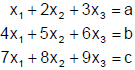will have no solutions or have an infinite number of solution.

Sol.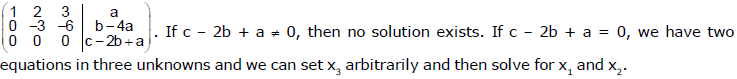J. INVERSE OF A MATRIX
(i) Singular & Non Singular Matrix : A square matrix A is said to be singular or non–singular according as |A| is zero or non–zero respectively.

Ex.31 Show that every skew-symmetric matrix of odd order is singular.

Sol.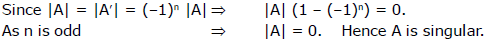(ii) Cofactor Matrix & Adjoint Matrix : Let A = [aij]n be a square matrix. The matrix obtained by
replacing each element of A by corresponding cofactor is called as cofactor matrix of A,
denoted as cofactor A. The transpose of cofactor matrix of A is called as adjoint of A, denoted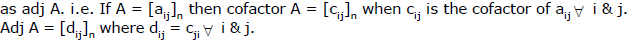(iii) Properties of Cofactor A and adj A :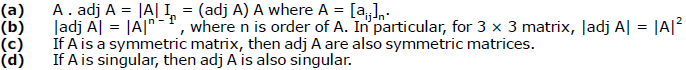(iv) Inverse of A Matrix (Reciprocal Matrix) : Let A be a non–singular matrix. Then the matrix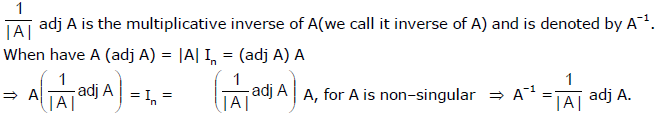Remarks :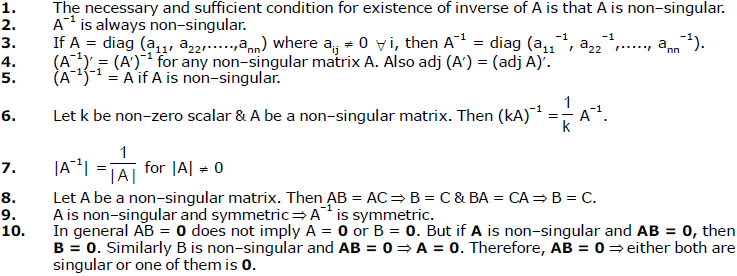Characteristic Polynomial & Characteristic Equation : Let A be a square matrix. Then the
polynomial |A – xI| is called as characteristic polynomial of A & the equation |A – xI| = 0 is called as
characteristic equation A.

Remark : Every square matrix A satisfies its characteristic equation (Cayley – Hamilton Theorem).
i.e.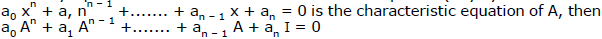Ex.32 Find the adjoint of the matrix A =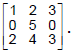Sol.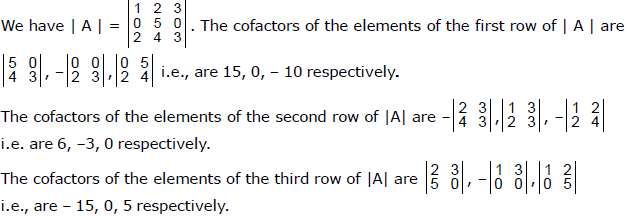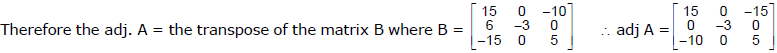Ex.33 If A and B are square matrices of the same order, then adj (AB) = adj B. adj A.

Sol.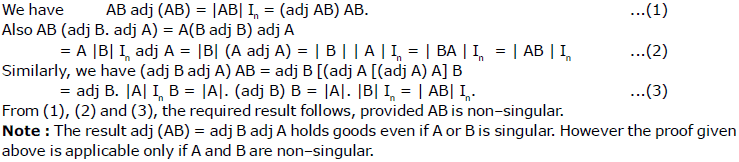Ex.34 If A be an n-square matrix and B be its adjoint, then show that Det (AB + KIn) = [Det (A) + K]n, where K is a scalar quantity.

Sol.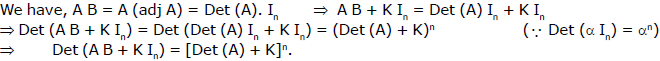Ex.35 If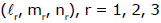be the direction cosines of three mutually perpendicular lines referred to an orthogonal Cartesian co–ordinate system, then prove that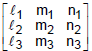is an orthogonal matrix.

Sol.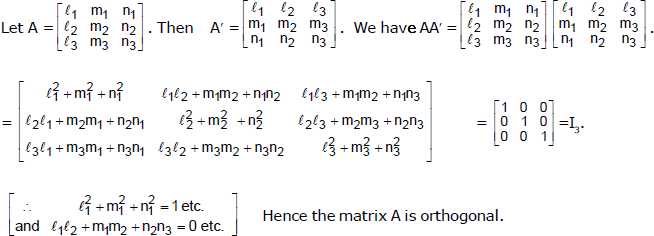Ex.36 Obtain the characteristic equation of the matrix A =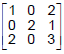and verify that it is satisfied y A and hence find its inverse.

Sol.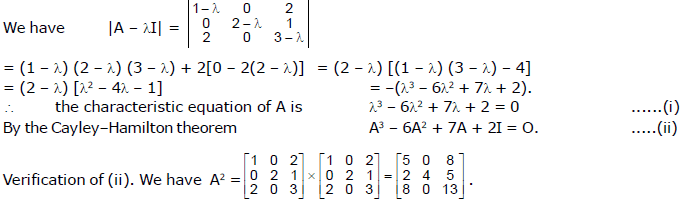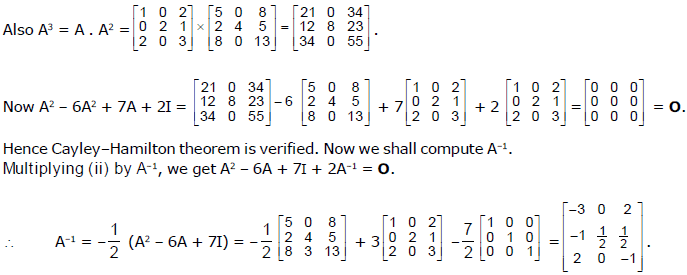Ex.37 Find the inverse of the matrix A =Sol.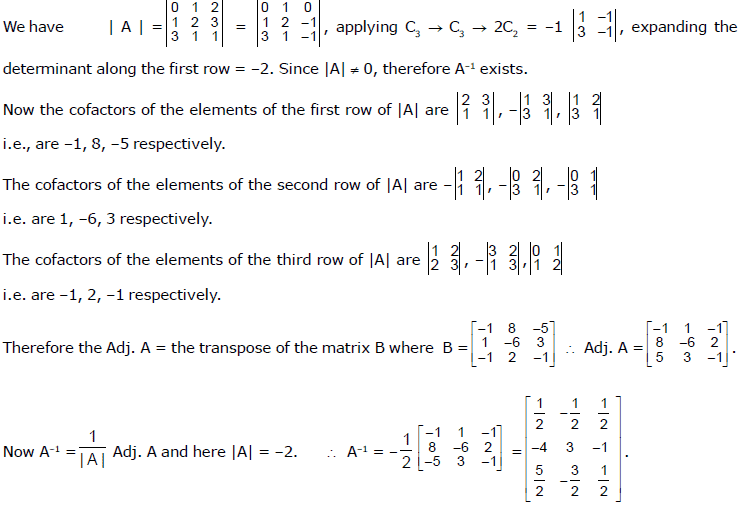Ex.38 If a non-singular matrix A is symmetric, show that A–1 is also symmetric.

Sol.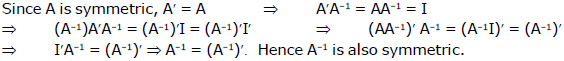Offer running on EduRev: Apply code STAYHOME200 to get INR 200 off on our premium plan EduRev Infinity!

## Mathematics (Maths) Class 12

209 videos|222 docs|124 tests

,

,

,

,

,

,

,

,

,

,

,

,

,

,

,

,

,

,

,

,

,

;